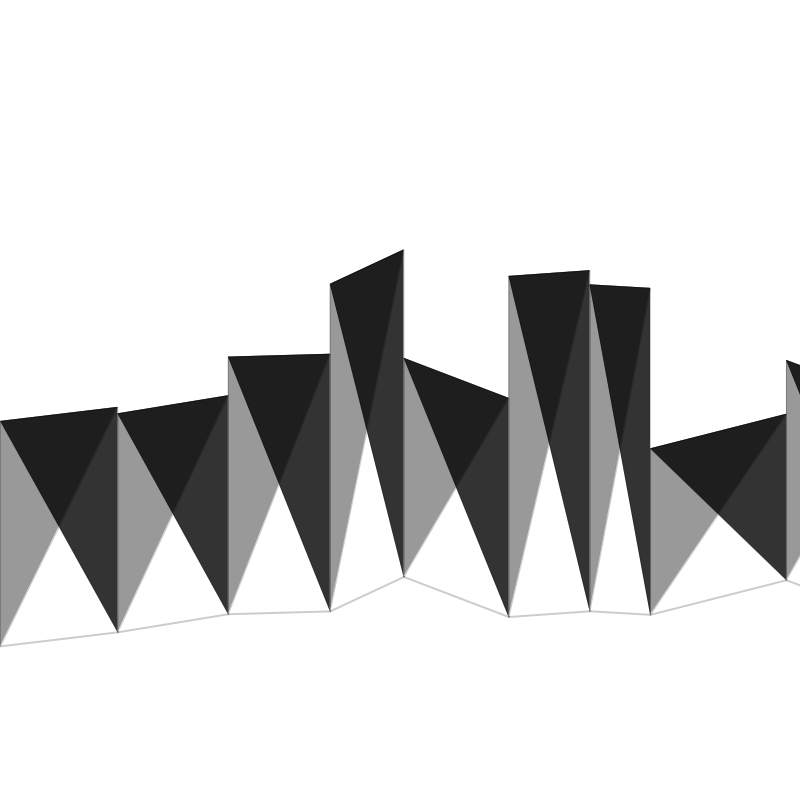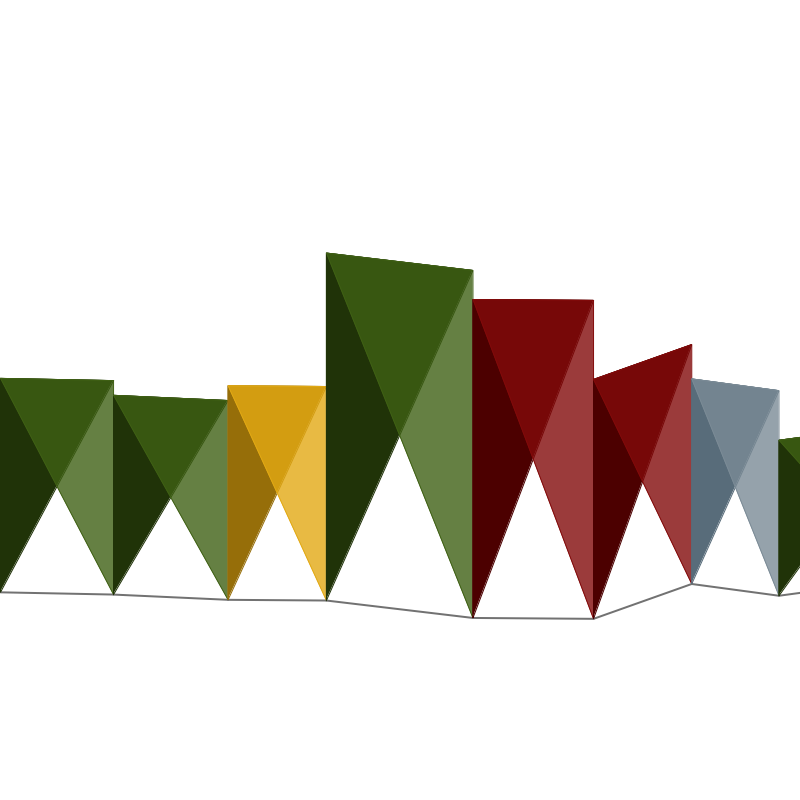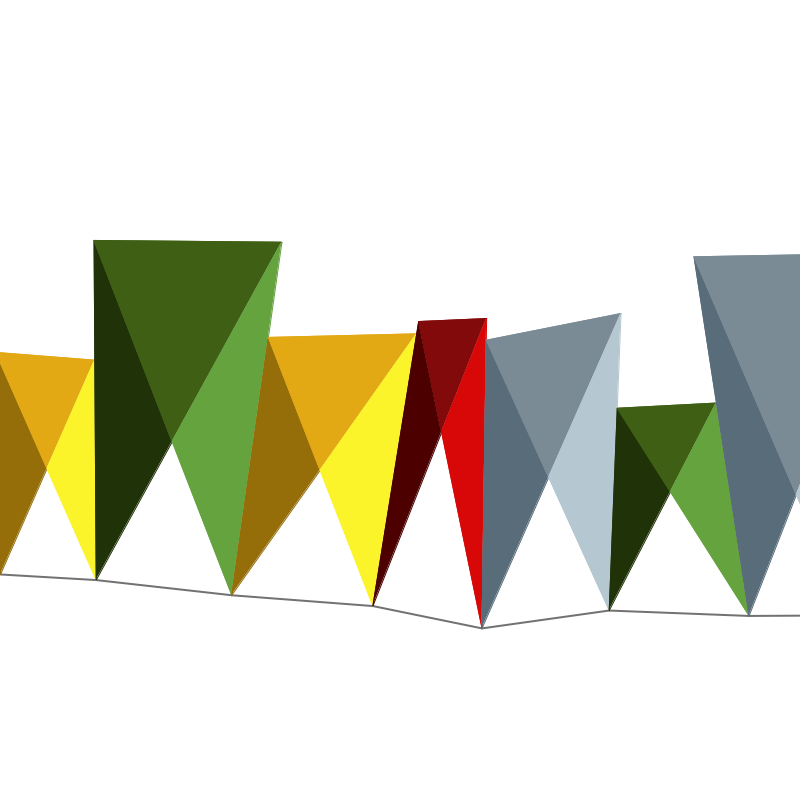# Randomization Homework

In these short examples, I’ll show how I would solve this week’s homework using vectors.Here’s the most basic sketch, in black and white, that demonstrates how to use vectors and perlin noise to calculate the positions of the triangles.See example codeSee example code

In the examples above, there are 2 problems:

1. The colors in the original work is not created by overlaying two triangles with different opacity 2. The shapes are going straight up in the air, and not rotating.

This is solved in this (more complicated sketch) by using 3 different colors and 3 triangles, and calculating their placement using the `Rune.Polygon` and `centroid()`.See example code